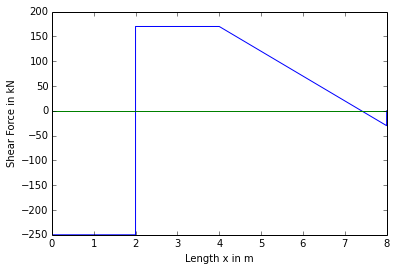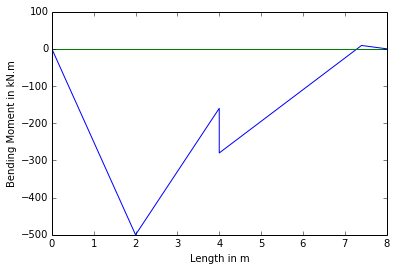# Chapter No.4:Shearing Force And Bending Moment¶

## Problem no 4.4.1,Page No.89¶

In :
import math
%matplotlib  inline

#Initilization of variables

F_B=-10 #KN #Force at pt B
F_D=-20 #KN #Force at pt D
w_CB=5 #KN/m #u.d.l at CB
w_AE=40 #KN/m #u.d.l at AE
L_ED=L_CB=2 #m #Length of ED & CB
L_CD=1 #m #Length of CD
L_AE=3 #m #Length of AE
L=8 #m #span of beam

#Calculations

#Shear Force Calculations

#Shear Force at B
V_B=F_B #KN

#Shear Force at C
V_C=F_B-(w_CB*L_CB)

#Shear Force at D
V_D1=V_C
V_D2=V_C+F_D

#Shear Force at E
V_E=V_D2

#Shear Force at A
V_A=V_D-(w_AE*L_AE)

#Bending Moment Calculations

#Bending Moment at B
M_B=0

#Bending Moment at C
M_C=F_B*L_CB-w_CB*L_CB**2*2**-1

#Bending Moment at D
M_D=F_B*(L_CB+L_CD)-w_CB*L_CB*(L_CB*2**-1+L_CD)

#Bending Moment at E
M_E=F_B*(L_CB+L_CD+L_ED)-w_CB*L_CB*(L_CB*2**-1+L_CD+L_ED)+F_D*L_ED

#Bending Moment at A
M_A=F_B*L-w_CB*L_CB*(L_CB*2**-1+L_CD+L_ED+L_AE)+F_D*(L_AE+L_ED)-w_AE*(L_AE**2*2**-1)

#Result
print "The Shear Force and Bending Moment Diagrams are the results"

#Plotting the Shear Force Diagram

X1=[0,L_CB,L_CB+L_DC,L_CB+L_DC,L_CB+L_DC+L_ED,L_CB+L_DC+L_ED+L_AE,L_CB+L_DC+L_ED+L_AE]
Y1=[V_B,V_C,V_D1,V_D2,V_E,V_A,0]
Z1=[0,0,0,0,0,0,0]
plt.plot(X1,Y1,X1,Z1)
plt.xlabel("Length x in m")
plt.ylabel("Shear Force in kN")
plt.show()

#Plotting the Bendimg Moment Diagram

Y2=[M_B,M_C,M_D,M_E,M_A]
X2=[0,L_CB,L_CB+L_DC,L_CB+L_DC+L_ED,L_CB+L_DC+L_ED+L_AE]
Z2=[0,0,0,0,0]
plt.plot(X2,Y2)
plt.xlabel("Lenght in m")
plt.ylabel("Bending Moment in kN.m")
plt.show()

The Shear Force and Bending Moment Diagrams are the results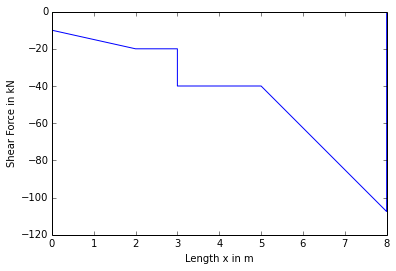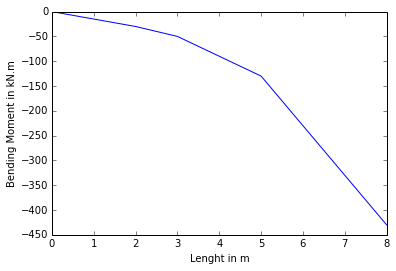## Problem no 4.4.2,Page No.90¶

In :
import math
%matplotlib  inline

#Initilization of variables
w_CB=1 #KN/m #u.d.l on Length CB
F_D=2 #KN #Pt Load at D
L_CB=2 #m #Length of CB

#Calculations

#Shear Force at B
V_B=0 #KN

#Shear Force at C
V_C=-(w_CB*L_CB)

#Shear Force at D
V_D1=V_C
V_D2=V_C-F_D

#Shear Force at A
V_A=V_D2

#Bending Moment Calculations

#Bending Moment at B
M_B=0

#Bending Moment at C
M_C=-w_CB*L_CB**2*2**-1

#Bending Moment at D
M_D=-w_CB*L_CB*(L_CB*2**-1+L_DC)

#Bending Moment at A

#Result
print "The Shear Force and Bending Moment Diagrams are the results"

#Plotting the Shear Force Diagram

Y1=[0,V_C,V_D1,V_D2,V_A,0]
Z1=[0,0,0,0,0,0]
plt.plot(X1,Y1,X1,Z1)
plt.xlabel("Length x in m")
plt.ylabel("Shear Force in kN")
plt.show()

#Plotting the Bendimg Moment Diagram

Y2=[M_B,M_C,M_D,M_A]
Z2=[0,0,0,0]
plt.plot(X2,Y2)
plt.xlabel("Length in m")
plt.ylabel("Bending Moment in kN.m")
plt.show()

The Shear Force and Bending Moment Diagrams are the results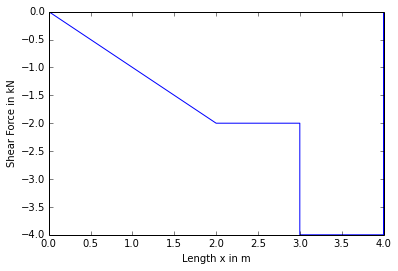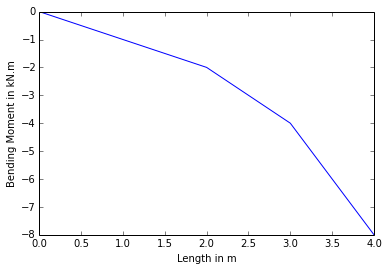## Problem no 4.4.3,Page No.91¶

In :
import math
%matplotlib  inline

#Initilization of variables
AC=5000 #N/m #u.v.l
L_AB=4 #m #Length of AB

#Calculations

#Consider a section at Distance x from B
#DB=x
#By similar triangles (triangle ABC and BDE) we get

#Shear Force at x
#F_x=-DB*DE*2**-1
#After substituting values in above equation we get
#F_x=625*x**2

#shear Force at B where x=0
V_B=0

#shear Force at A where x=L_AB=4
V_A=625*L_AB**2

#Bending Moment Calculation

#M_x=DB*DE*DB*3**-1*2**-1
#Substituting values in above equation we get
#M_x=-625*x**3*3**-1

#Bending Moment at B where x=0
M_B=0

#Bending Moment at A where x=L_AB=4
M_A=-625*L_AB**3*3**-1

#Result
print "The Shear Force and Bending Moment Diagrams are the results"

#Plotting the Shear Force Diagram

X1=[0,L_AB]
Y1=[V_B,V_A]
Z1=[0,0]
plt.plot(X1,Y1,X1,Z1)
plt.xlabel("Length x in m")
plt.ylabel("Shear Force in kN")
plt.show()

#Plotting the Bendimg Moment Diagram

Y2=[M_B,M_A]
X2=[0,L_AB]
Z2=[0,0]
plt.plot(X2,Y2)
plt.xlabel("Length in m")
plt.ylabel("Bending Moment in kN.m")
plt.show()

The Shear Force and Bending Moment Diagrams are the results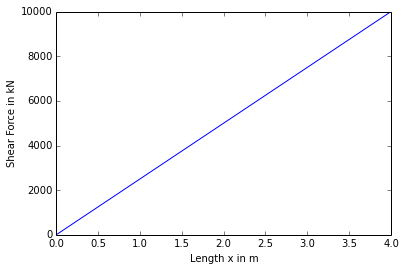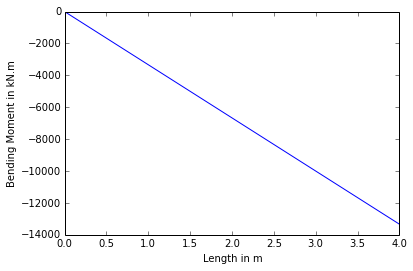## Problem no 4.4.4,Page No.92¶

In :
import math
%matplotlib  inline

#Initilization of variables
F_C=F_D=F_E=30 #KN #Pt Load at C,D,E respectively
L_AE=L_ED=L_DC=1.5 #m #Length of AE,ED,DC respectively
L_CB=0.5 #m #Length of CB
L_AC=4.5 #m #Length of AC
w=10 #KN/m #u.d.l
L=5 #m #Length of beam

#Calculations

#Shear Force Calculations

#Shear Force at B
V_B=0 #KN

#Shear Force at C
V_C1=-w_CB*L_CB
V_C2=-w*L_CB-F_C #KN

#Shear Force at D
V_D1=-w*(L_DC+L_CB)-F_C*L_DC
V_D2=-w*(L_DC+L_CB)-F_C-F_D #KN

#Shear Force at E
V_E1=-w*(L_DC+L_CB+L_ED)-F_C*(L_DC+L_ED)
V_E2=-F_C-F_D-F_E-w*(2*L_ED+L_CB)

#Shear Force at A
V_A=-w*L-F_C-F_D-F_E

#Bending Moment Calculations

#Bending Moment at B
M_B=0

#Bending Moment at C
M_C=-w*L_CB**2*2**-1

#Bending Moment at D
M_D=-w*(L_DC+L_CB)**2*2**-1-F_C*L_DC

#Bending Moment at E
M_E=-w*(L_DC+L_CB+L_ED)**2*2**-1-F_C*(L_ED+L_DC)-F_D*L_ED

#Bending Moment at A

#Result
print "The Shear Force and Bending Moment Diagrams are the results"

#Plotting the Shear Force Diagram

X1=[0,L_CB,L_CB,L_CB+L_DC,L_CB+L_DC,L_CB+L_DC+L_ED,L_CB+L_DC+L_ED,L_CB+L_DC+L_ED+L_AE,L_CB+L_DC+L_ED+L_AE]
Y1=[V_B,V_C1,V_C2,V_D1,V_D2,V_E1,V_E2,V_A,0]
Z1=[0,0,0,0,0,0,0,0,0]
plt.plot(X1,Y1,X1,Z1)
plt.xlabel("Length x in m")
plt.ylabel("Shear Force in kN")
plt.show()

#Plotting the Bendimg Moment Diagram

X2=[0,L_CB,L_CB+L_DC,L_CB+L_DC+L_ED,L_CB+L_DC+L_ED+L_AE,L_CB+L_DC+L_ED+L_AE]
Y2=[M_B,M_C,M_D,M_E,M_A,0]
Z2=[0,0,0,0,0,0]
plt.plot(X2,Y2)
plt.xlabel("Length in m")
plt.ylabel("Bending Moment in kN.m")
plt.show()

The Shear Force and Bending Moment Diagrams are the results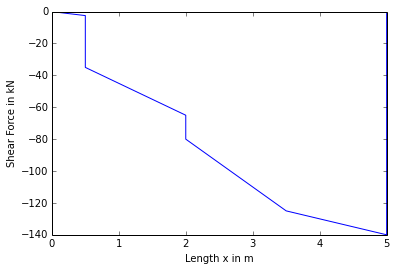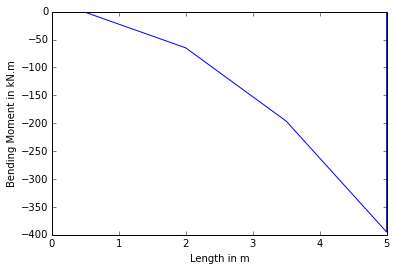## Problem no 4.4.5,Page No.93¶

In :
import math
%matplotlib  inline

#Initilization of variables

w1=30 #KN/m #u.d.l on L_CB
F_C=120 #KN #Pt Load at C
L_DC=L_CB=2 #m #Length of DC and CB respectively
L_AB=L=8 #m #Length of beam

#Calculations

#Let R_A & R_B be the reactions at A & B
#R_A+R_B=380

#Taking Moment at A

#After Rearranging the terms we get
R_A=380-R_B

#Shear Force Calculations

#Shear Force at B
V_B=R_B

#Shear Force at C
V_C1=-w1*L_CB+R_B
V_C2=R_B-w1*L_CB-F_C

#Shear Force at D
V_D=V_C2

#Shear Force at A

#Point of contraflexure
#Let E be the point EB=x
#Shear Force at E
#V_E=0=R_B-F_C-w1*L_CB-w2*(L_EB-L_DC-L_CB)
L_EB=-((-R_B+F_C+w1*L_CB)*w2**-1-L_DC-L_CB)
V_E=0

#Bending Moment Calculations

#Bending Moment at B
M_B=0

#Bending Moment at C
M_C=R_B*L_CB-w1*L_CB**2*2**-1

#Bending Moment at D
M_D=R_B*(L_CB+L_DC)-w1*L_CB*(L_CB*2**-1+L_DC)-F_C*L_DC

#Bending Moment at A
M_A=0

#Bending Moment at E
L_ED=L_EB-(L_DC+L_CB) #m #Length of ED
M_E=-w1*L_CB*(L_ED+L_DC+L_CB*2**-1)-F_C*(L_DC+L_ED)+R_B*L_EB

#Result
print "The Shear Force and Bending Moment Diagrams are the results"

#Plotting the Shear Force Diagram

Y1=[V_B,V_C1,V_C2,V_D,V_A,0]
Z1=[0,0,0,0,0,0]
plt.plot(X1,Y1,X1,Z1)
plt.xlabel("Length x in m")
plt.ylabel("Shear Force in kN")
plt.show()

#Plotting the Bendimg Moment Diagram

Y2=[M_B,M_C,M_D,M_E,M_A]
Z2=[0,0,0,0,0]
plt.plot(X2,Y2)
plt.xlabel("Length in m")
plt.ylabel("Bending Moment in kN.m")
plt.show()

The Shear Force and Bending Moment Diagrams are the results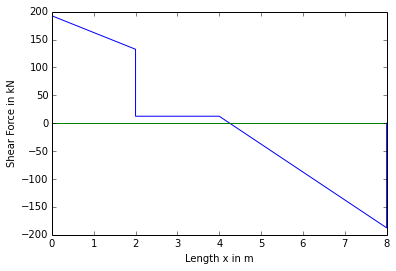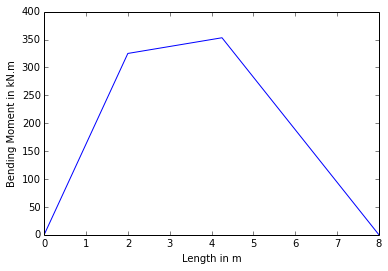## Problem no 4.4.6,Page No.95¶

In :
import math
%matplotlib  inline

#Initilization of variables
F_C=100 #KN #Pt Load at C
F_E=50 #KN #Pt Load at E
w=20 #KN/m
L_AE=L_ED=L_DC=L_CB=2 #m #Length of AE,ED,DC,CB respectively
L=8 #m #Length of Beam

#Calculations

#Let R_A & R_B be the reactions at A & B
#R_A+R_B=190

#Taking Moment at A
#M_A=-R_B*L+F_C*(3*L_AE)+w*L_DC*(L_DC*2**-1+2*L_ED)+F_E*L_AE=0
R_B=(F_C*(3*L_AE)+w*L_DC*(L_DC*2**-1+2*L_ED)+F_E*L_AE)*L**-1
R_A=190-R_B

#Shear Force Calculations

#Shear Force at B
V_B=R_B

#Shear Force at C
V_C1=R_B
V_C2=R_B-F_C

#Shear Force at D
V_D=V_C2-w*L_DC

#Shear Force at E
V_E1=V_D
V_E2=V_D-F_E

#Shear Force at A
V_A=V_E2

#Point of contraflexure
#Let F be the point BF=x
#Shear Force at F
#V_F=R_B-F_C-w*(L_BF-L_CB)
L_FB=-((-R_B+F_C)*w**-1-L_CB)
V_F=0

#Bending Moment Calculations

#Bending Moment at B
M_B=0

#Bending Moment at C
M_C=R_B*L_CB

#Bending Moment at D
M_D=R_B*(L_CB+L_DC)-F_C*L_DC-w*L_DC**2*2**-1

#Bending Moment at E
M_E=R_B*(L_CB+L_DC+L_ED)-F_C*(L_ED+L_DC)-w*L_DC*(L_DC*2**-1+L_ED)

#Bending Moment at A
M_A=R_B*(L_ED+L_DC+L_AE+L_CB)-F_C*(L_ED+L_DC+L_AE)-w*L_DC*(L_DC*2**-1+L_ED+L_AE)-F_E*L_AE

#Bending Moment at F
L_FC=L_BF-L_CB
M_F=R_B*L_FB-F_C*L_FC-w*L_FC**2*2**-1
L_DF=L_DC-L_FC

#Result
print "The Shear Force and Bending Moment Diagrams are the results"

#Plotting the Shear Force Diagram

X1=[0,L_CB,L_CB,L_CB+L_DC,L_CB+L_DC+L_ED,L_CB+L_DC+L_ED,L_CB+L_DC+L_ED+L_AE]
Y1=[V_B,V_C1,V_C2,V_D,V_E1,V_E2,V_A]
Z1=[0,0,0,0,0,0,0,]
plt.plot(X1,Y1,X1,Z1)
plt.xlabel("Length x in m")
plt.ylabel("Shear Force in kN")
plt.show()

#Plotting the Bendimg Moment Diagram

Y2=[M_B,M_C,M_F,M_D,M_E,M_A]
Z2=[0,0,0,0,0,0]
plt.plot(X2,Y2)
plt.xlabel("Length in m")
plt.ylabel("Bending Moment in kN.m")
plt.show()

The Shear Force and Bending Moment Diagrams are the results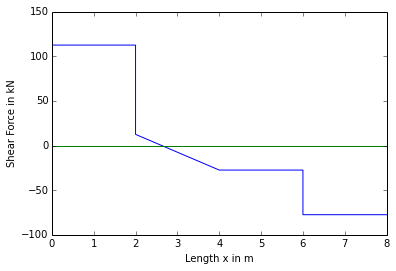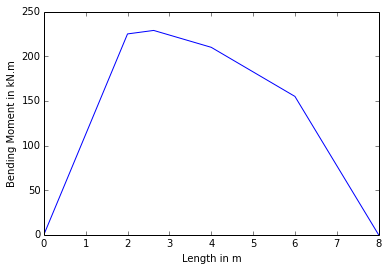## Problem no 4.4.7,Page No.96¶

In :
import math
%matplotlib  inline

#Initilization of variables
w=20 #KN/m #u.d.l on Length  CB
F_D= 50 #KN #Pt Load at D
L_CB=5 #m #Length of CB
L_DC=3 #M #Length of DC
L=10 #m #Length of Beam

#Calculations

theta=arctan(4*3**-1)*(180*pi**-1)
F_DV=F_D*sin(theta*pi*180**-1) #Force at Pt D vertically
F_DH=F_D*cos(theta*pi*180**-1) #Force at pt D horizontally

#Let R_A & R_B be the reactions at A & B
#R_A+R_B=140

#Taking Moment at A
R_A=140-R_B

#Shear Force Calculations

#Shear Force at B
V_B=R_B

#Shear Force at C
V_C=V_B-w*L_CB

#Shear Force at D
V_D1=V_C
V_D2=V_C-F_DV

#Shear Force at A
V_A=V_D

#Pt of Contraflexure
#Let E be the pt And BE=x
#V_E=0=R_B-w*x
x=L_BE=R_B*w**-1

#Bending Moment Calculations

#Bending Moment at B
M_B=0

#Bending Moment at C
M_C=R_B*L_CB-w*L_CB**2*2**-1

#Bending Moment at D
M_D=R_B*(L_CB+L_DC)-w*L_CB*(L_CB*2**-1+L_DC)

#Bending Moment at A

#Bending Moment at E
M_E=R_B*L_BE-w*L_BE**2*2**-1

#Result
print "The Shear Force and Bending Moment Diagrams are the results"

#Plotting the Shear Force Diagram

Y1=[V_B,V_C,V_D1,V_D2,V_A]
Z1=[0,0,0,0,0]
plt.plot(X1,Y1,X1,Z1)
plt.xlabel("Length x in m")
plt.ylabel("Shear Force in kN")
plt.show()

#Plotting the Bendimg Moment Diagram

Y2=[M_B,M_E,M_C,M_D,M_A]
Z2=[0,0,0,0,0,0]
plt.plot(X2,Y2)
plt.xlabel("Length in m")
plt.ylabel("Bending Moment in kN.m")
plt.show()

The Shear Force and Bending Moment Diagrams are the results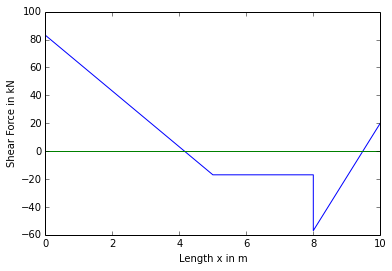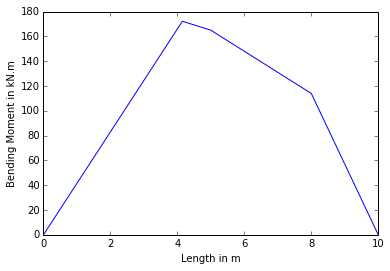## Problem no 4.4.8,Page No.97¶

In :
import math
%matplotlib  inline

#Initilization of variables
F_C=150 #KN #Pt LOad at C
w=300 #KN #u.v.l
L=6 #m #Length of beam
L_AE=L_DC=L_CB=1 #m #Lengthof AE,DC,CB
L_ED=3 #m #Length of ED
L_Ed=2 #m
L_dD=1 #m

#Calculations

#Let R_A & R_B be the reactions at A & B
#R_A+R_B=450

#Taking Moment at A
#M_A=0=R_B*L-F_C*(L_CD+L_ED+L_AE)-w*(2*3**-1*L_ED+L_AE)
R_B=(F_C*(L_DC+L_ED+L_AE)+w*(2*3**-1*L_ED+L_AE))*L**-1
R_A=450-R_B

#Shear Force Calculations

#Shear Force at B
V_B=R_B

#Shear Force at C
V_C1=R_B
V_C2=R_B-F_C

#Shear Force at D
V_D=V_C2

#Shear Force at E
V_E=V_D-w

#Shear Force at A
V_A=V_E

#Pt of contraflexure
#Let F be the pt and EF=x
w1=w*2*3**-1

#V_F=0=-R_B+200*x*3**-1*x*2**-1
#After substituting values and simplifying further we get
L_EF=x=(R_A*3*100**-1)**0.5

#Bending Moment Calculations

#Bending Moment at B
M_B=0

#Bending Moment at C
M_C=R_B*L_CB

#Bending Moment at D
M_D=R_B*(L_CB+L_DC)-F_C*L_DC

#Bending Moment at E
M_E=R_B*(L_CB+L_DC+L_ED)-F_C*(L_DC+L_ED)-w*L_Ed

#Bending Moment at A
M_A=0

#Bending Moment at F
M_F=R_A*(L_AE+L_EF)-200*x*3**-1*x*2**-1*x*3**-1

L_FD=L_ED-L_EF

#Result
print "The Shear Force and Bending Moment Diagrams are the results"

#Plotting the Shear Force Diagram

X1=[0,L_CB,L_CB,L_CB+L_CD,L_CB+L_CD+L_ED,L_CB+L_CD+L_ED+L_AE,L_CB+L_CD+L_ED+L_AE]
Y1=[V_B,V_C1,V_C2,V_D,V_E,V_A,0]
Z1=[0,0,0,0,0,0,0]
plt.plot(X1,Y1,X1,Z1)
plt.xlabel("Length x in m")
plt.ylabel("Shear Force in kN")
plt.show()

#Plotting the Bendimg Moment Diagram

X2=[0,L_CB,L_CB+L_DC,L_FD+L_DC+L_CB,L_CB+L_DC+L_ED,L_CB+L_DC+L_ED+L_AE]
Y2=[M_B,M_C,M_D,M_F,M_E,M_A]
Z2=[0,0,0,0,0,0]
plt.plot(X2,Y2)
plt.xlabel("Length in m")
plt.ylabel("Bending Moment in kN.m")
plt.show()

The Shear Force and Bending Moment Diagrams are the results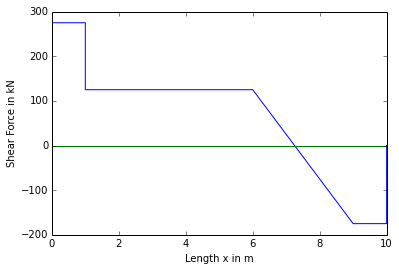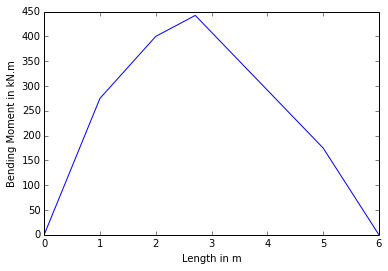## Problem no 4.4.9,Page No.99¶

In :
import math
%matplotlib  inline

#Initilization of variables
M_C=40 #KNM #Moment at Pt C
L=10 #m #Length of beam
L_CB=5 #m #Length of CB
L_DC=1 #m #Length of DC

#Calculations

#Let R_A & R_B be the reactions at A & B
#R_A+R_B=80

#Taking Moment at A
R_A=80-R_B

#Shear Force Calculations

#Shear Force at B
V_B=R_B

#Shear Force at C
V_C=V_B

#Shear Force at D
V_D=V_C

#Shear Force at A

#Pt of contraflexure
#Let E be the pt and BE=x
#V_E=0=R_B-w*(L_BE-L_DC-L_CB)
L_BE=x=R_B*w**-1+L_DC+L_CB

#Bending Moment Calculations

#Bending Moment at B
M_B=0

#Bending Moment at C
M_C1=R_B*L_CB
M_C2=M_C1-M_C

#Bending Moment at D
M_D=R_B*(L_CB+L_DC)-M_C

#Bending Moment at A

#Bending Moment at E
L_ED=L_BE-(L_DC+L_CB)
M_E=R_B*L_BE-M_C-w*L_ED**2*2**-1

#Result
print "The Shear Force and Bending Moment Diagrams are the results"

#Plotting the Shear Force Diagram

Y1=[V_B,V_C,V_D,V_A,0]
Z1=[0,0,0,0,0]
plt.plot(X1,Y1,X1,Z1)
plt.xlabel("Length x in m")
plt.ylabel("Shear Force in kN")
plt.show()

#Plotting the Bendimg Moment Diagram

Y2=[M_B,M_C1,M_C2,M_D,M_E,M_A]
Z2=[0,0,0,0,0,0]
plt.plot(X2,Y2,X2,Z2)
plt.xlabel("Length in m")
plt.ylabel("Bending Moment in kN.m")
plt.show()

The Shear Force and Bending Moment Diagrams are the results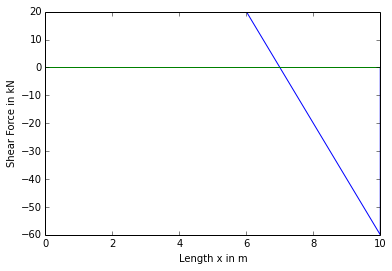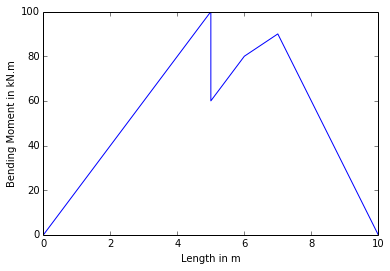## Problem no 4.4.10,Page No.100¶

In :
import math
%matplotlib  inline

#Initilization of variables
F_D=20 #KN #Pt Load at D
M_C=240 #KNm #moment at Pt C
L_DC=L_CB=2 #m #Length of DC and CB
L=8 #m #Length of Beam

#Calculations

#Calculations

#Let R_A & R_B be the reactions at A & B
#R_A+R_B=60

#Taking Moment at A
R_A=60-R_B

#Shear Force Calculations

#Shear Force at B
V_B=R_B

#Shear Force at C
V_C=V_B

#Shear Force at D
V_D1=V_B
V_D2=V_D1-F_D

#Shear Force at A

#Bending Moment Calculations

#Bending Moment at B
M_B=0

#Bending Moment at C
M_C1=R_B*L_CB
M_C2=M_C+R_B*L_CB

#Bending Moment at D
M_D=R_B*(L_DC+L_CB)+M_C

#Bending Moment at A

#Result
print "The Shear Force and Bending Moment Diagrams are the results"

#Plotting the Shear Force Diagram

Y1=[V_B,V_C,V_D1,V_D2,V_A]
Z1=[0,0,0,0,0]
plt.plot(X1,Y1,X1,Z1)
plt.xlabel("Length x in m")
plt.ylabel("Shear Force in kN")
plt.show()

#Plotting the Bendimg Moment Diagram

Y2=[M_B,M_C1,M_C2,M_D,M_A,0]
Z2=[0,0,0,0,0,0]
plt.plot(X2,Y2,X2,Z2)
plt.xlabel("Length in m")
plt.ylabel("Bending Moment in kN.m")
plt.show()

The Shear Force and Bending Moment Diagrams are the results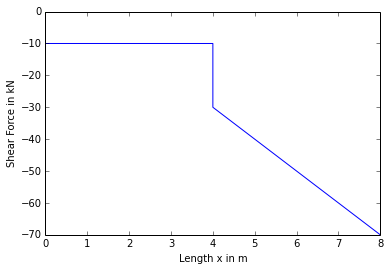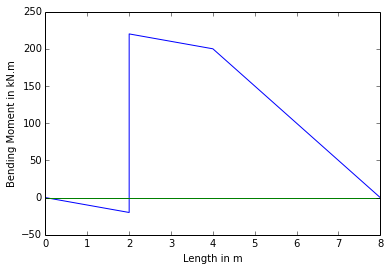## Problem no 4.4.11,Page No.101¶

In :
import math
%matplotlib  inline

#Initilization of variables
F_C=5 #KN #Force at C
w=2 #KNm #u.d.l on beam
L_BC=3 #m #Length of BC
L_AB=6 #m #Length of AB
L=9 #m #Length of Beam

#Calculations

#Let R_A & R_B be the reactions at A & B
#R_A+R_B=23

#Taking Moment at A
#M_A=0=F_C*L-R_B*L_AB+w*L**2*2**-1
R_B=-(-F_C*L-w*L**2*2**-1)*L_AB**-1
R_A=23-R_B

#Shear Force Calculations

#Shear Force at C
V_C1=0
V_C2=-F_C

#Shear Force at B
V_B=V_C2-w*L_BC**2*2**-1

#Shear Force at A
V_A=F_C*L+R_B*L_AB-w*L**2*2**-1

#Pt of contraflexure
#Let D be the pt And L_AD=x

#Bending Moment Calculations

#Bending Moment at C
M_C=0

#Bending Moment at B
M_B=-F_C*L_BC-w*L_BC**2*2**-1

#Bending Moment at A
M_A=-F_C*L-w*L**2*2**-1+R_B*L_AB

#Bending Moment at D
L_DB=L_DC-L_BC

#Result
print "The Shear Force and Bending Moment Diagrams are the results"

#Plotting the Shear Force Diagram

X1=[0,L_BC,L_BC+L_AB,L_BC+L_AB]
Y1=[V_C,V_B,V_A,0]
Z1=[0,0,0,0]
plt.plot(X1,Y1,X1,Z1)
plt.xlabel("Length x in m")
plt.ylabel("Shear Force in kN")
plt.show()

#Plotting the Bendimg Moment Diagram

X2=[0,L_BC,L_BC+L_DB,L_BC+L_AB]
Y2=[M_C,M_B,M_D,M_A]
Z2=[0,0,0,0]
plt.plot(X2,Y2,X2,Z2)
plt.xlabel("Length in m")
plt.ylabel("Bending Moment in kN.m")
plt.show()

#The Bending moment in book is incorrect

The Shear Force and Bending Moment Diagrams are the results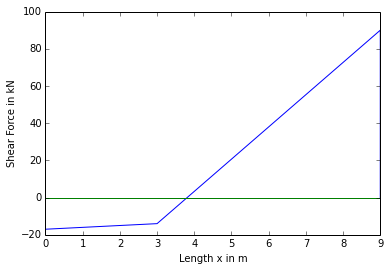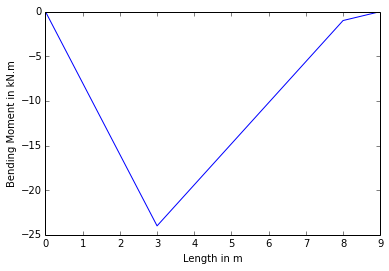## Problem no 4.4.12,Page No.102¶

In :
import math
%matplotlib  inline

#Initilization of variables
F_C=5 #KN #Pt Load at C
F_D=4 #KN #Pt Load at D
L_BC=1.25 #m #Length of BC
L_DB=1 #m #Length of DB
w=2 #KN/m #u.d.l
L=5.25 #m #Length of beam

#Calculations

#Let R_A & R_B be the reactions at A & B
#R_A+R_B=15

#Taking Moment at A
R_A=15-R_B

#Shear Force Calculations

#Shear Force at C
V_C=-F_C

#Shear Force at B
V_B1=V_C
V_B2=V_C+R_B

#Shear Force at D
V_D1=V_B2
V_D2=V_B2-F_D

#Shear Force at A

#Pt of contraflexure
#Let E be the pt and BE=x
#V_E=0=-F_C+R_B-F_D-w*(L_BE-L_DB)
L_BE=x=-((F_C-R_B+F_D)*w**-1-L_DB)

#Bending Moment Calculations

#Bending Moment at C
M_C=0

#Bending Moment at B
M_B=-F_C*L_BC

#Bending Moment at D
M_D=-F_C*(L_DB+L_BC)-R_B*L_DB

#Bending Moment at A

#Bending Moment at E
L_ED=L_BE-L_DB
M_E=-F_C*(L_BC+L_BE)+R_B*L_BE-F_D*(L_BE-L_DB)-w*(L_BE-L_DB)**2*2**-1

#Result
print "The Shear Force and Bending Moment Diagrams are the results"

#Plotting the Shear Force Diagram

Y1=[V_C,V_B1,V_B2,V_D1,V_D2,V_A,0]
Z1=[0,0,0,0,0,0,0]
plt.plot(X1,Y1,X1,Z1)
plt.xlabel("Length x in m")
plt.ylabel("Shear Force in kN")
plt.show()

#Plotting the Bendimg Moment Diagram

Y2=[M_C,M_B,M_D,M_E,M_A]
Z2=[0,0,0,0,0]
plt.plot(X2,Y2,X2,Z2)
plt.xlabel("Length in m")
plt.ylabel("Bending Moment in kN.m")
plt.show()

The Shear Force and Bending Moment Diagrams are the results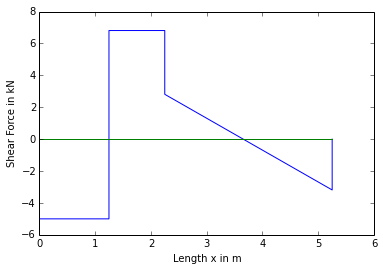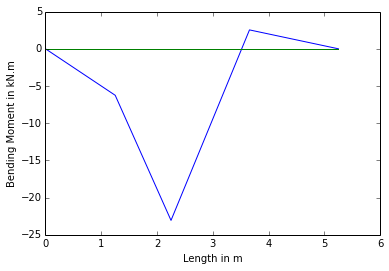## Problem no 4.4.13,Page No.103¶

In :
import math
%matplotlib  inline

#Initilization of variables
F_E=20 #KN #Pt Load at E
F_C=30 #KN #Pt Load at C
F_B=60 #KN #Pt Load at B
L_AB=L_BC=L_CD=1.5 #m #Length of AB,BC,CD respectively
L_DE=2.5 #m #Length od DE
L=7 #m #Length of beam
w=30 #KN/m

#Calculations

#LEt R_A and R_D be the reactions at A and D
#R_A+R_D=245

#Taking moment at A
R_A=245-R_D

#Shear Force Calculations

#Shear Force at C
V_E1=0
V_E2=-F_E

#Shear Force at D
V_D1=V_E2
V_D2=V_E2+R_D

#Shear Force at C
V_C1=V_D2
V_C2=V_D2-F_C-w*L_CD

#Shear Force at B
V_B1=V_C2
V_B2=-F_E+R_D-F_C-w*(L_BC+L_CD)-F_B

#Shear Force at A

#Pt of contraflexure
#Let F be the pt and EF=x
#V_F=-F_E-F_C+R_D-w*L_FE+w*L_DE
L_FE=-(F_E+F_C-R_D-w*L_DE)*w**-1
L_FD=L_FE-L_DE
L_FC=L_FE-L_CD-L_DE

#Bending Moment Calculations

#Bending Moment at E
M_E=0

#Bending Moment at D
M_D=-F_E*L_DE

#Bending Moment at C
M_C=-F_E*(L_CD+L_DE)+R_D*L_CD-w*L_CD**2*2**-1

#Bending Moment at F
M_F=-w*L_FD**2*2**-1-F_C*L_FC+R_D*L_FD-F_E*L_FE

#Bending Moment at B
M_B=-F_E*(L_DE+L_CD+L_BC)-F_C*L_BC+R_D*(L_CD+L_BC)-w*(L_BC+L_CD)**2*2**-1

#Bending Moment at A

#Bending Moment at F
M_F=-F_E*L_FE+R_D*L_FD-F_C*L_FC-w*L_FD**2*2**-1

#Pt of contraflexure
#Let G be the pt and GE=y
#M_G=-F_E*L_GE+R_D*(L_GE-L_DE)-F_C*(L_GE-L_DE)**2*2**-1
#After substituting values and further simplifying we get
#y**2-12.9+29.35=0
a=1
b=-12.9
c=29.35

X=b**2-4*a*c

y1=(-b+X**0.5)*(2*a)**-1
y2=(-b-X**0.5)*(2*a)**-1

#Result
print "The Shear Force and Bending Moment Diagrams are the results"

#Plotting the Shear Force Diagram

X1=[0,0,L_DE,L_DE,L_DE+L_CD,L_DE+L_CD,L_DE+L_CD+L_BC,L_DE+L_CD+L_BC,L_DE+L_CD+L_BC+L_AB,L_DE+L_CD+L_BC+L_AB]
Y1=[V_E1,V_E2,V_D1,V_D2,V_C1,V_C2,V_B1,V_B2,V_A,0]
Z1=[0,0,0,0,0,0,0,0,0,0]
plt.plot(X1,Y1,X1,Z1)
plt.xlabel("Length x in m")
plt.ylabel("Shear Force in kN")
plt.show()

#Plotting the Bendimg Moment Diagram

X2=[0,L_DE,L_DE+L_CD,L_DE+L_CD+L_FC,L_DE+L_CD+L_BC,L_DE+L_CD+L_BC+L_AB]
Y2=[M_E,M_D,M_C,M_F,M_B,M_A]
Z2=[0,0,0,0,0,0]
plt.plot(X2,Y2,X2,Z2)
plt.xlabel("Length in m")
plt.ylabel("Bending Moment in kN.m")
plt.show()

The Shear Force and Bending Moment Diagrams are the results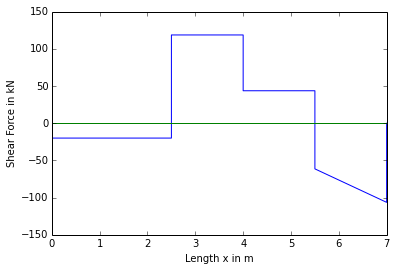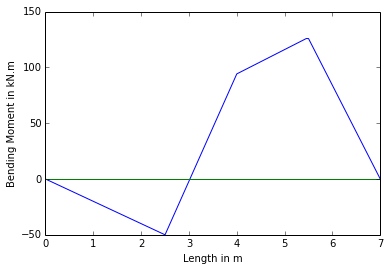## Problem no 4.4.14,Page No.105¶

In :
import math
%matplotlib  inline

#Initilization of variables
L_DE=L_BC=2.5 #m #Length of DE & BC
L_CD=L_AB=5 #m #Length of CD & AB
F_C=F_B=80 #KN #Pt Load at C & B
w1=16 #KN/m #u.d.l on L_DE
w2=10 #KN/m #u.d.l on L_AB
L=10 #m #Length of beam

#Calculations

#LEt R_A and R_D be the reactions at A and D
#R_A+R_D=250

#Taking moment at A
#M_A=0=w1*L_DE*(L_DE*2**-1+L_CD+L_BC+L_AB)-R_D*(L_CD+L_BC+L_AB)+F_C*(L_BC+L_AB)+F_C*(L_BC+L_AB)+F_B*L_AB+w2*L_AB**2*2**-1
R_D=-(-w1*L_DE*(L_DE*2**-1+L_CD+L_BC+L_AB)-F_C*(L_BC+L_AB)-F_B*(L_AB)-w2*L_AB**2*2**-1)*(L_CD+L_BC+L_AB)**-1
R_A=250-R_D

#Shear Force Calculations

#Shear Force at E
V_E=0

#Shear Force at D
V_D1=-w1*L_DE
V_D2=-w1*L_DE+R_D

#Shear Force at C
V_C1=V_D2
V_C2=V_D2-F_C

#Shear Force at B
V_B1=V_C2
V_B2=V_C2-F_B

#Shear Force at A
V_A1=V_B2-w2*L_AB
V_A2=0

#Bending Moment Calculations

#Bending Moment at E
M_E=0

#Bending Moment at D
M_D=-w1*L_DE**2*2**-1

#Bending Moment at C
M_C=R_D*L_CD-w1*L_DE*(L_DE*2**-1+L_CD)

#Bending Moment at B
M_B=-w1*L_DE*(L_DE*2**-1+L_CD+L_BC)+R_D*(L_CD+L_BC)-F_C*L_BC

#Bending Moment at A
M_A=-w1*L_DE*(L_DE*2**-1+L_CD+L_BC+L_AB)+R_D*(L_CD+L_BC+L_AB)-F_C*(L_BC+L_AB)-F_B*L_AB-w2*L_AB**2*2**-1

#Result
print "The Shear Force and Bending Moment Diagrams are the results"

#Plotting the Shear Force Diagram

X1=[0,L_FE,L_FE,L_DE+L_CD,L_DE+L_CD,L_DE+L_CD+L_BC,L_DE+L_CD+L_BC,L_DE+L_CD+L_BC+L_AB,L_DE+L_CD+L_BC+L_AB]
Y1=[V_E,V_D1,V_D2,V_C1,V_C2,V_B1,V_B2,V_A1,V_A2]
Z1=[0,0,0,0,0,0,0,0,0]
plt.plot(X1,Y1,X1,Z1)
plt.xlabel("Length x in m")
plt.ylabel("Shear Force in kN")
plt.show()

#Plotting the Bendimg Moment Diagram

X2=[0,L_DE,L_DE+L_CD,L_DE+L_CD+L_BC,L]
Y2=[M_E,M_D,M_C,M_B,M_A]
Z2=[0,0,0,0,0]
plt.plot(X2,Y2,X2,Z2)
plt.xlabel("Length in m")
plt.ylabel("Bending Moment in kN.m")
plt.show()

The Shear Force and Bending Moment Diagrams are the results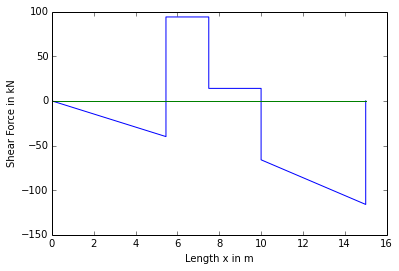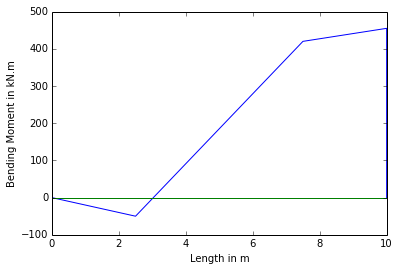## Problem no 4.4.15,Page No.105¶

In :
import math
%matplotlib  inline

#Initilization of variables
L=8 #m #Length of beam
w=300 #KN #u.d.l

#Calculations

#Let R_A and R_C be the reactions at A and C
#R_A+R_C=300

#Taking moment at A
#LEt x be the distance from Pt B L_CB=x
#R_C*(L-L_CB)=300*L*2**-1
#R_C=1200*(8-x)**-1
#After substituting values and further simplifying we get
#R_A=300-R_C
#R_A=1200-300*x*(8-x)**-1

#B.M at D

#Now substituting value of R_A we get
#M_D=4*1200-300*x*(8-x)**-1-300=0

#Further on simplification we get
L_CB=x=600*225**-1

R_C=1200*(8-x)**-1
R_A=(1200-300*x)*(8-x)**-1

#Pt of contraflexure
#Let E be the pt and BE=y
#V_E=0=-R_A*2**-1*L_BE+R_C
L_BE=R_C*(R_A*2**-1)**-1
L_AE=L-L_BE
L_AC=L-L_CB
L_EC=L_BE-L_CB

#Shear Force at B
V_B=0

#Shear Force at C
V_C1=-w
V_C2=-V_C1+R_C

#Shear Force at A
V_A=-w+R_C

#B.M at C
M_C=-w*L_CB

#B.M at E
M_E=-R_A*L_AE+w*L_AE

#B.M at A
M_A=0

#Result
print "The Shear Force and Bending Moment Diagrams are the results"

#Plotting the Shear Force Diagram

X1=[0,L_CB,L_CB,L_CB+L_AC,L_CB+L_AC]
Y1=[V_B,V_C1,V_C2,V_A,0]
Z1=[0,0,0,0,0]
plt.plot(X1,Y1,X1,Z1)
plt.xlabel("Length x in m")
plt.ylabel("Shear Force in kN")
plt.show()

#Plotting the Bendimg Moment Diagram

X2=[0,L_CB,L_CB+L_EC,L_CB+L_AC]
Y2=[M_B,M_C,M_E,M_A]
Z2=[0,0,0,0]
plt.plot(X2,Y2,X2,Z2)
plt.xlabel("Length in m")
plt.ylabel("Bending Moment in kN.m")
plt.show()

The Shear Force and Bending Moment Diagrams are the results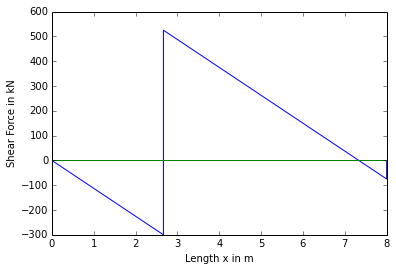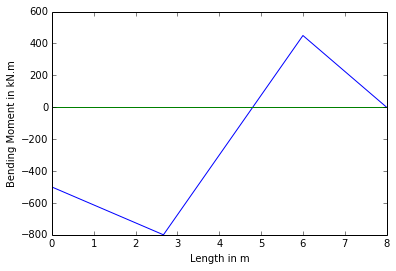## Problem no 4.4.16,Page No.107¶

In :
import math
%matplotlib  inline

#Initilization of variables
F_C=250 #KN #Pt LOad at C
M_D=120 #KNM #moment at Pt D
L_DB=L_BC=2 #m #Length of DB & BC
L=8 #m #Length of beam

#Calculations

#LEt R_A and R_D be the reactions at A and D
#R_A+R_D=450

#Taking moment at A
R_D=450-R_B

#Shear Force Calculations

#Shear Force at C
V_C=-F_C

#Shear Force at B
V_B1=V_C
V_B2=R_B-F_C

#Shear Force at D
V_D=V_B2

#Shear Force at A

#Pt of contralfexure
#Let E be the pt and CE=x
#V_E=0=-F_C+R_B-w*(L_EC-L_DB-L_BC)
L_EC=-((+F_C-R_B)*w**-1-L_DB-  L_BC)
L_ED=L_EC-L_DB-L_BC

#Bending Moment Calculations

#Bending Moment at C
M_C=0

#Bending Moment at B
M_B=-F_C*L_BC

#Bending Moment at D
M_D1=-F_C*(L_BC+L_DB)+R_B*L_DB
M_D2=M_D1-M_D

#Bending Moment at E
M_E=-F_C*L_EC+R_B*(L_ED+L_DB)-w*L_ED**2*2**-1-M_D

#Bending Moment at A
M_A=0

#Pt of contraflexure
#Let F be the pt and CF=y
#M_F=0=- F_C*L_FC+R_B*(L_FC-L_BC)-M_D-w*(L_FC-L_DB-L_BC)
#After substituting values and further simplifying we get equation as
#y**2-14.8*y+54.5=0

a=1
b=-14.8
c=54.4

X=b**2-4*a*c

y1=(-b+X**0.5)*(2*a)**-1
y2=(-b-X**0.5)*(2*a)**-1

#From above two equations y2 is taken into consideration

#Result
print "The Shear Force and Bending Moment Diagrams are the results"

#Plotting the Shear Force Diagram

Y1=[V_C,V_B1,V_B2,V_D,V_A,0]
Z1=[0,0,0,0,0,0]
plt.plot(X1,Y1,X1,Z1)
plt.xlabel("Length x in m")
plt.ylabel("Shear Force in kN")
plt.show()

#Plotting the Bendimg Moment Diagram


The Shear Force and Bending Moment Diagrams are the results